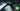# Hackerrank | Solution of Grading Students in Golang

September 16th, 2018
|

In this post we will solve question grading students from hackerrank in golang. Let's dive right into it.

# Problem Statement

The question can be found at this link. The problem statement states that we need to round up grades of students in a class based on some given conditions.

Couple of things to note here is

• We have to round up the grade to next higher multiple of 5.
• For the rounding, the difference between the number and next higher multiple should be less than 3.
• If the marks is less than 38, no rounding will occur, as the student will still fail, given the condition that 40 is passing marks.

# Challenges

• Choose our preferred language as golang on hackerrank. The moment we do that, we get some 50-60 lines of code which are very unfamiliar to someone who is new to language.
• Working with multiple. Little bit of maths, nothing very tough.

# Solution

This is the template you get on hackerrank for this problem statement.

``````    package main

import (
"bufio"
"fmt"
"io"
"os"
"strconv"
"strings"
)

/*
* Complete the gradingStudents function below.
*/
/*
*/

}

func main() {

outputFile, err := os.Create(os.Getenv("OUTPUT_PATH"))
checkError(err)

defer outputFile.Close()

writer := bufio.NewWriterSize(outputFile, 1024 * 1024)

checkError(err)
n := int32(nTemp)

checkError(err)
}

for resultItr, resultItem := range result {
fmt.Fprintf(writer, "%d", resultItem)

if resultItr != len(result) - 1 {
fmt.Fprintf(writer, "\n")
}
}

fmt.Fprintf(writer, "\n")

writer.Flush()
}

if err == io.EOF {
return ""
}

return strings.TrimRight(string(str), "\r\n")
}

func checkError(err error) {
if err != nil {
panic(err)
}
}``````
• First go ahead and delete everything in the pre-existing template.
• Let's write down our main wrapper function.
``````package main
import "fmt"

func main(){

}``````

In this question we will be given the length of the array of marks and the array it self. However we don't need to store array values for any future reference, we can safely store it in a temporary variable. Let's go ahead and declare our variables and take the array length as input.

``````    ...
var n,t int
fmt.Scan(&n)``````

Now we need to loop over the length of array times and take our inputs.

``````    ...
for i:=0;i<n;i++{
fmt.Scan(&t)

// write logic here

}``````

Now it's time for the real thing, the logic. So there are two parts to it. First one being if the marks is less than 38, second everything else. In the case where marks are less than 38, it is pretty simple no rounding up is required and we will just print the value as it is. Then we have the second case, unfortunately this one has two cases too. I promise these two are the last. So if the number and next higher multiple of five has a difference less than 3 then we will round up the number, else we will just print the number as it is. If we rephrase the last statement a bit, if the difference between the number and the lower multiple of five is greater than 2 we will round up the number, else print as it is. So now the conditions are decided, how would we calculate the difference between the number and lower multiple of five. Just take a remainder from five. If we think a bit about it, `81` gives a remainder `1`, which is difference of `81-80`, and so on. So let's fill in our logic.

``````	...

if t<38{
fmt.Println(t)
}else{
if t % 5 > 2 {
// round our number
fmt.Println(((t/5)+1)*5)
}else {
fmt.Println(t)
}
}

...``````

The block above replaces the code comment that we put earlier inside for loop. The `if` block is straight forward, if the number is less than 38, we print the number as it is. In the else we are calculating the difference as discussed and if the difference is greater than 2, we are rounding up else printing it as it is. The only alien here is the line below `// round our number` comment. There we are actually rounding our number and printing it. Let's discuss it. So first we divide the number by 5. It gives the number which when multiplied by five will produce the lower multiple of five. Now we add one to it and at last we multiply five to get the actual number. Allow me to explain with an example. Let's take `84`. `84/5` will give `16` and not `16.something` because both numerator and denominator are integers. Now we add One to it. `16+1 = 17` and multiply it by 5, `17*5 = 85`. Hurray!!! we have our rounded number. I hope I was able to clear it up. So now our complete code should look like

``````package main

import "fmt"

func main(){
var n,t int
fmt.Scan(&n)

for i:=0;i<n;i++{
fmt.Scan(&t)
if t<38{
fmt.Println(t)
}else{
if t % 5 > 2 {
// round our number
fmt.Println(((t/5)+1)*5)
}else {
fmt.Println(t)
}
}
}
}``````

Let's review what are the highlights to take away from this question. To get a lower multiple of five of a particular number just divide that number by five and again multiply it by five. If you think about this and this alone, every thing was based on this logic. This is it for this one, complete source code for this post can be found on my Github Repo. Will see you in the next one.